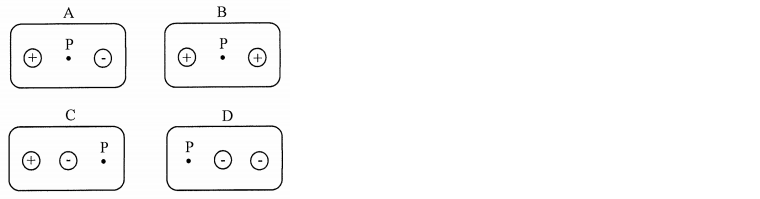# Problem: If a negative charge (-Q) is placed at point P in the above diagram, which situation minimizes the electric potential energy?A) Situation AB) Situation BC) Situation CD) Situation D

###### FREE Expert Solution
97% (331 ratings)
###### Problem Details

If a negative charge (-Q) is placed at point P in the above diagram, which situation minimizes the electric potential energy?

A) Situation A

B) Situation B

C) Situation C

D) Situation DFrequently Asked Questions

What scientific concept do you need to know in order to solve this problem?

Our tutors have indicated that to solve this problem you will need to apply the Electric Potential Energy concept. You can view video lessons to learn Electric Potential Energy. Or if you need more Electric Potential Energy practice, you can also practice Electric Potential Energy practice problems.

How long does this problem take to solve?

Our expert Physics tutor, Juan took 4 minutes and 46 seconds to solve this problem. You can follow their steps in the video explanation above.